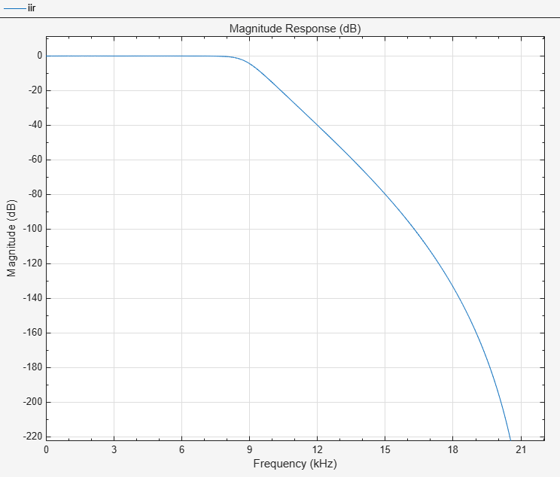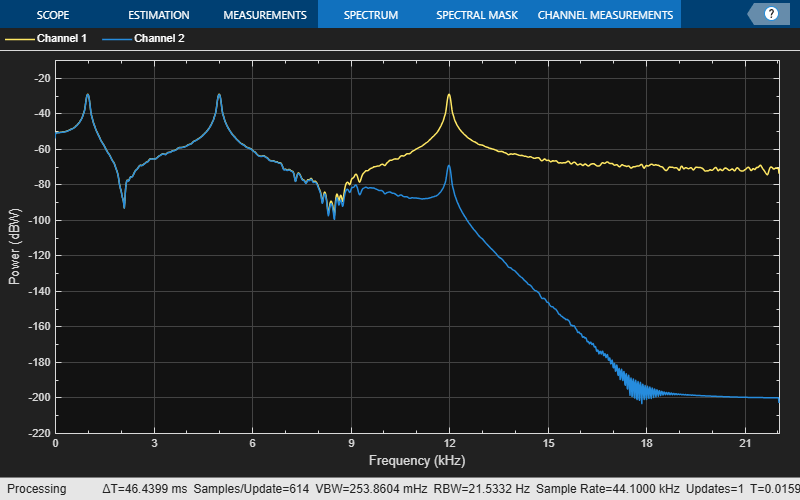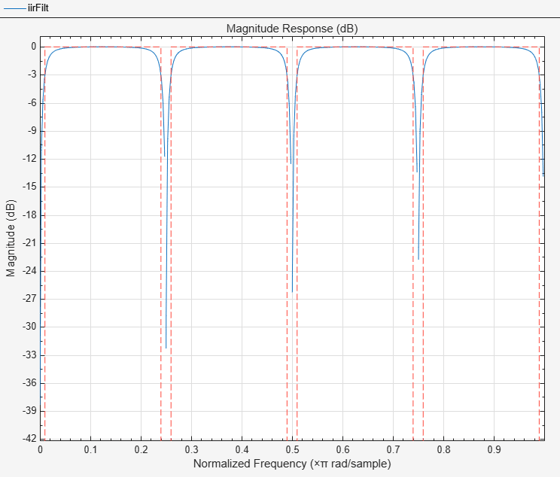# dsp.IIRFilter

Infinite impulse response (IIR) filter

## Description

The `dsp.IIRFilter` System object™ filters each channel of the input using the specified filter. You can specify the filter to have a `'Direct form I'`, ```'Direct form I transposed'```, `'Direct form II'`, or ```'Direct form II transposed'``` structure.

Use the Numerator and Denominator properties to specify the coefficients of the filter numerator and denominator coefficients. In addition to these coefficients, you can also specify nonzero initial filter states through the InitialConditions property.

To filter a signal using an IIR filter:

1. Create the `dsp.IIRFilter` object and set its properties.

2. Call the object with arguments, as if it were a function.

## Creation

### Syntax

``iir = dsp.IIRFilter``
``iir = dsp.IIRFilter(Name,Value)``

### Description

example

````iir = dsp.IIRFilter` creates an infinite impulse response (IIR) filter System object that independently filters each channel of the input over time using a specified IIR filter implementation.```

example

````iir = dsp.IIRFilter(Name,Value)` creates an IIR filter object with each specified property set to the specified value. Enclose each property name in single quotes.Example: iir = dsp.IIRFilter('Structure','Direct form I');```

## Properties

expand all

Unless otherwise indicated, properties are nontunable, which means you cannot change their values after calling the object. Objects lock when you call them, and the `release` function unlocks them.

If a property is tunable, you can change its value at any time.

IIR filter structure, specified as `'Direct form I'`, `'Direct form I transposed'`, `'Direct form II'`, or `'Direct form II transposed'`.

Numerator coefficients, specified as a row vector.

Example: [```0.0296 0.1775 0.4438 0.5918 0.4438 0.1775 0.0296```]

Tunable: Yes

Data Types: `single` | `double` | `int8` | `int16` | `int32` | `int64` | `uint8` | `uint16` | `uint32` | `uint64`
Complex Number Support: Yes

Denominator coefficients, specified as a row vector.

Example: [```1.0000 -0.0000 0.7777 -0.0000 0.1142 -0.0000 0.0018```]

Tunable: Yes

Data Types: `single` | `double` | `int8` | `int16` | `int32` | `int64` | `uint8` | `uint16` | `uint32` | `uint64`
Complex Number Support: Yes

Initial conditions of the filter states, specified as one of the following:

• scalar –– The object initializes all delay elements in the filter to the scalar value.

• vector –– The length of the vector equals the number of delay elements in the filter. Each vector element specifies a unique initial condition for the corresponding delay element. The object applies the same vector to each channel of the input signal.

• matrix –– The number of rows in the matrix must equal the number of delay elements in the filter. The number of columns in the matrix must equal the number of channels in the input. Each element specifies a unique initial condition for the corresponding delay element in the corresponding channel.

The number of filter states equals max(N,M) – 1, where N is the number of poles, and M is the number of zeros.

Tunable: Yes

#### Dependencies

This property applies only when you set the Structure property to ```'Direct form II'``` or `'Direct form II transposed'`.

Data Types: `single` | `double` | `int8` | `int16` | `int32` | `int64` | `uint8` | `uint16` | `uint32` | `uint64`
Complex Number Support: Yes

Initial conditions of the filter states on the side of the filter structure with the zeros, specified as one of the following:

• scalar –– The object initializes all delay elements on the zeros side in the filter to the scalar value.

• vector –– The length of the vector equals the number of delay elements on the zeros side in the filter. Each vector element specifies a unique initial condition for the corresponding delay element on the zeros side. The object applies the same vector of initial conditions to each channel of the input signal.

• matrix –– The number of rows in the matrix must equal the number of delay elements on the zeros side in the filter. The number of columns in the matrix must equal the number of channels in the input signal. Each element specifies a unique initial condition for the corresponding delay element on the zeros side in the corresponding channel.

The number of filter states equals max(N,M) – 1, where N is the number of poles, and M is the number of zeros, respectively.

Tunable: Yes

#### Dependencies

This property applies only when you set the Structure property to ```'Direct form I'``` or `'Direct form I transposed'`.

Data Types: `single` | `double` | `int8` | `int16` | `int32` | `int64` | `uint8` | `uint16` | `uint32` | `uint64`
Complex Number Support: Yes

Initial conditions of the filter states on the side of the filter structure with the poles, specified as one of the following:

• scalar –– The object initializes all delay elements on the poles side in the filter to the scalar value.

• vector –– The length of the vector equals the number of delay elements on the poles side in the filter. Each vector element specifies a unique initial condition for the corresponding delay element on the poles side. The object applies the same vector of initial conditions to each channel of the input signal.

• matrix –– The number of rows in the matrix must equal the number of delay elements on the poles side in the filter. The number of columns in the matrix must equal the number of channels in the input signal. Each element specifies a unique initial condition for the corresponding delay element on the poles side in the corresponding channel.

The number of filter states equals max(N,M) – 1, where N is the number of poles, and M is the number of zeros, respectively.

Tunable: Yes

#### Dependencies

This property applies only when you set the Structure property to ```'Direct form I'``` or `'Direct form I transposed'`.

Data Types: `single` | `double` | `int8` | `int16` | `int32` | `int64` | `uint8` | `uint16` | `uint32` | `uint64`
Complex Number Support: Yes

#### Fixed-Point Properties

Select the rounding mode for fixed-point operations.

Overflow action for fixed-point operations, specified as one of the following:

• `'Wrap'` –– The object wraps the result of its fixed-point operations.

• `'Saturate'` –– The object saturates the result of its fixed-point operations.

For more details on overflow actions, see overflow mode for fixed-point operations.

State data type, specified as one of the following:

• `'Same as input'` –– The state data type is same as the input data type.

• `'Custom'` –– The state output data type is an autosigned numeric type through the CustomStateDataType property.

State word and fraction lengths, specified as an autosigned numeric type with a word length of 16 and a fraction length of 15.

#### Dependencies

This property applies only when you set StateDataType to `'Custom'`.

Data type of numerator coefficients, specified as one of the following:

• `'Same word length as input'` –– The word length of the numerator coefficients is the same as the input word length. The fraction length is chosen to give the best possible precision.

• `'Custom'` –– The data type of the numerator coefficients is the autosigned numeric type specified by the CustomNumeratorCoefficientsDataType property.

Word and fraction lengths of the numerator coefficients, specified as an autosigned numeric type with a word length of 16 and a fraction length of 15.

#### Dependencies

This property applies only when you set NumeratorCoefficientsDataType to `'Custom'`.

Data type of the denominator coefficients, specified as one of the following:

• `'Same word length as input'` –– The word length of the denominator coefficients is the same as that of the input word length. The fraction length is chosen to give the best possible precision.

• `'Custom'` –– The data type of the denominator coefficients is the autosigned numeric type specified by the CustomDenominatorCoefficientsDataType property.

Word and fraction lengths of denominator coefficients, specified as an autosigned numeric type with a word length of 16 and a fraction length of 15.

#### Dependencies

This property applies only when you set DenominatorCoefficientsDataType to `'Custom'`.

Data type of the output of a product operation in the numerator polynomial of the IIR filter, specified as one of the following:

• `'Full precision'` –– The object computes the numerator product output data type using the full-precision rules. These rules provide the most accurate fixed-point numerics. No quantization occurs. Bits are added, as needed, to ensure that no roundoff or overflow occurs.

• `'Same as input'` –– The product output data type is the same as the input data type.

• `'Custom'` –– The product output data type is the custom numeric type specified by the CustomNumeratorProductDataType property. The rounding method and the overflow action are specified by the RoundingMethod and OverflowAction properties.

Numerator product word and fraction lengths, specified as an autosigned numeric type with a word length of 32 and a fraction length of 30.

#### Dependencies

This property applies only when you set NumeratorProductDataType to `'Custom'`.

Data type of the output of a product operation in the denominator polynomial of the IIR filter, specified as one of the following:

• `'Full precision'` –– The object computes the denominator product output data type using the full-precision rules. These rules provide the most accurate fixed-point numerics. No quantization occurs. Bits are added, as needed, to ensure that no roundoff or overflow occurs.

• `'Same as input'` –– The product output data type is the same as the input data type.

• `'Custom'` –– The product output data type is custom numeric type specified by the CustomDenominatorProductDataType property. The rounding method and the overflow action are specified by the RoundingMethod and OverflowAction properties.

Denominator product word and fraction lengths, specified as an autosigned numeric type with a word length of 32 and a fraction length of 30.

#### Dependencies

This property applies only when you set DenominatorProductDataType to `'Custom'`.

Data type of the output of an accumulation operation in the numerator polynomial of the IIR filter, specified as one of the following:

• `'Full precision'` –– The object computes the numerator accumulator data type using the full-precision rules. These rules provide the most accurate fixed-point numerics. No quantization occurs. Bits are added, as needed, to ensure that no roundoff or overflow occurs.

• `'Same as input'` –– The accumulator data type is the same as the input data type.

• `'Same as product'` –– The accumulator data type is the same as the product output data type.

• `'Custom'` –– The accumulator data type is the custom numeric type specified by the CustomNumeratorAccumulatorDataType property. The rounding method and the overflow action are specified by the RoundingMethod and OverflowAction properties.

Numerator accumulator word and fraction lengths, specified as an autosigned numeric type with a word length of 32 and a fraction length of 30.

#### Dependencies

This property applies only when you set NumeratorAccumulatorDataType to `'Custom'`.

Data type of the output of an accumulation operation in the denominator polynomial of the IIR filter, specified as one of the following:

• `'Full precision'` –– The object computes the denominator accumulator data type using the full-precision rules. These rules provide the most accurate fixed-point numerics. No quantization occurs. Bits are added, as needed, to ensure that no roundoff or overflow occurs.

• `'Same as input'` –– The accumulator data type is the same as the input data type.

• `'Same as product'` –– The accumulator data type is the same as the product output data type.

• `'Custom'` –– The accumulator data type is the custom numeric type specified by the CustomDenominatorAccumulatorDataType property. The rounding method and the overflow action are specified by the RoundingMethod and OverflowAction properties.

Denominator accumulator word and fraction lengths, specified as an autosigned numeric type with a word length of 32 and a fraction length of 30.

#### Dependencies

This property applies only when you set DenominatorAccumulatorDataType to `'Custom'`.

Data type of the output of the `dsp.IIRFilter` object, specified as one of the following:

• `'Same as input'` –– The output data type is the same as the input data type.

• `'Full precision'` –– The object computes the output data type using the full-precision rules. These rules provide the most accurate fixed-point numerics. No quantization occurs. Bits are added, as needed, to ensure that no roundoff or overflow occurs.

• `'Custom'` –– The output data type is the custom numeric type specified by the CustomOutputDataType property. The rounding method and the overflow action are specified by the RoundingMethod and OverflowAction properties.

Output word and fraction lengths, specified as an autosigned numeric type with a word length of 16 and a fraction length of 15.

#### Dependencies

This property applies only when you set OutputDataType to `'Custom'`.

Multiplicand data type, specified as one of the following:

• `'Same as input'` –– The multiplicand data type is the same as the input data type.

• `'Custom'` –– The multiplicand data type is the autosigned numeric type specifies by the CustomMultiplicandDataType property.

Multiplicand output word and fraction lengths, specified as an autosigned numeric type with a word length of 16 and a fraction length of 15.

#### Dependencies

This property applies only when you set MultiplicandDataType to `'Custom'`.

## Usage

### Syntax

``iirOut = iir(input)``

### Description

example

````iirOut = iir(input)` filters the input signal using the specified filter to produce the filtered output. The System object filters each column of the input signal independently over time.```

### Input Arguments

expand all

Data input that is filtered, specified as a vector or matrix.

Example: `randn(34,24)`

Data Types: `single` | `double` | `int8` | `int16` | `int32` | `int64` | `fi`
Complex Number Support: Yes

### Output Arguments

expand all

Filtered output, returned as a vector or a matrix. The size, data type, and complexity of the outpout match that of the input.

Data Types: `single` | `double` | `int8` | `int16` | `int32` | `int64` | `fi`
Complex Number Support: Yes

## Object Functions

To use an object function, specify the System object as the first input argument. For example, to release system resources of a System object named `obj`, use this syntax:

`release(obj)`

expand all

 `freqz` Frequency response of filter `fvtool` Visualize frequency response of DSP filters `impz` Impulse response of discrete-time filter System object `phasez` Unwrapped phase response for filter
 `step` Run System object algorithm `release` Release resources and allow changes to System object property values and input characteristics `reset` Reset internal states of System object

For a list of filter analysis methods this object supports, type `dsp.IIRFilter.helpFilterAnalysis` in the MATLAB® command prompt. For the corresponding function reference pages, see Analysis Methods for Filter System Objects.

## Examples

collapse all

Filter a noisy sinusoidal signal using a lowpass butterworth IIR filter.

Note: If you are using R2016a or an earlier release, replace each call to the object with the equivalent step syntax. For example, `obj(x)` becomes `step(obj,x)`.

Input Signal

The input signal has three tones, 1 kHz, 5 kHz, and 12 kHz.

```Sine1 = dsp.SineWave('Frequency',1e3,'SampleRate',44.1e3); Sine2 = dsp.SineWave('Frequency',5e3,'SampleRate',44.1e3); Sine3 = dsp.SineWave('Frequency',12e3,'SampleRate',44.1e3);```

Filter Design

Use the `butter` function to design a 10th order lowpass Butterworth filter.

```N = 10; Fc = 0.4; [b,a] = butter(N,Fc);```

Create a `dsp.IIRFilter` object and assign the designed coefficients to the `Numerator` and the `Denominator` properties.

`iir = dsp.IIRFilter('Numerator',b,'Denominator',a);`

View the magnitude response of the filter. The cutoff frequency is at 0.4 rad/sample, which, with a sample rate of 44.1 kHz, translates to $0.4×44100/2$ or 8.82 kHz.

`fvtool(iir,'Fs',Sine1.SampleRate)`View the power spectrum of the input and output signal using the `Spectrum Analyzer`.

```sa = dsp.SpectrumAnalyzer('SampleRate',Sine1.SampleRate,'NumInputPorts',2,... 'PlotAsTwoSidedSpectrum',false,... 'OverlapPercent',80,'PowerUnits','dBW',... 'YLimits',[-220 -10]);```

Streaming

Add zero-mean white Gaussian noise with a standard deviation of 0.01 to the sum of sine waves. Filter the signal using the IIR filter.

The tones at 1 kHz and 5 kHz are unaffected since they fall in the passband. The frequency at 12 kHz is attenuated since it falls in the transition band of the filter.

```for i = 1:1000 input = Sine1()+Sine2()+Sine3()+0.01*randn(Sine1.SamplesPerFrame,1); output = iir(input); sa(input,output) end```Design a notching comb filter with 8 notches, and a notch bandwidth of 0.02 referenced to a -3 dB level.

Create a `comb` filter design specification object using the `fdesign.comb` function and specify these design parameters.

`combSpecs = fdesign.comb('notch','N,BW',8,0.02);`

Design the notching comb filter using the `design` function. The resulting filter is a `dsp.IIRFilter` System object™. For details on how to apply this filter on streaming data, refer to `dsp.IIRFilter`.

`iirFilt = design(combSpecs,'Systemobject',true)`
```iirFilt = dsp.IIRFilter with properties: Structure: 'Direct form II' Numerator: [0.8878 0 0 0 0 0 0 0 -0.8878] Denominator: [1 0 0 0 0 0 0 0 -0.7757] InitialConditions: 0 Show all properties ```

View magnitude response of the designed filter using `fvtool`.

`fvtool(iirFilt)`Watch now